#There are 366 different Starters of The Day, many to choose from. You will find below some starters on the topic of Mental Methods. A lesson starter does not have to be on the same topic as the main part of the lesson or the topic of the previous lesson. It is often very useful to revise or explore other concepts by using a starter based on a totally different area of Mathematics.

Main Page

### Mental Methods Starters: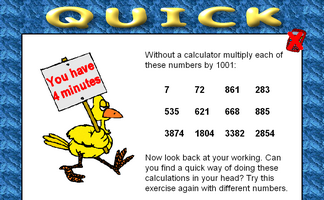Develop a quick way of mentally multiplying any number by 1001.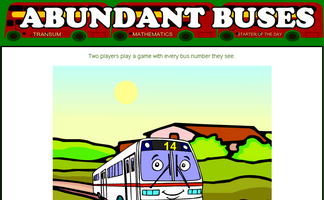A game based around the concept of factors and abundant numbers.Calculations appear on the screen every few seconds. This mental arithmetic starter provides pace to the start of the Maths lesson.This activity requires students to memorise fifteen numbers in a three by five grid.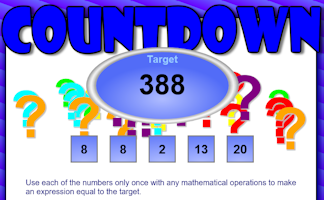How close can you get to the target by making a calculation from the five numbers given?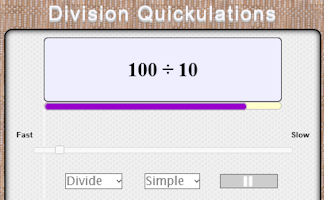Random division calculations appear on screen every few seconds.Practise multiplying and dividing by eleven in your head.Fit families onto eleven seater buses without splitting up the families.If each number in a sequence must be a factor or multiple of the previous number what is the longest sequence that can be made from the given numbers?Find four single digit numbers that multiply together to give 120. How many different ways are there of answering this question?Which of the numbers from one to twenty can you make with the digits 4, 5, 6 and 7?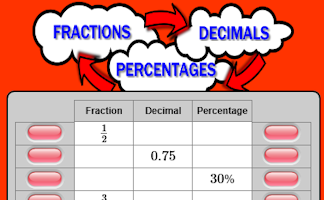Convert fractions to decimals, decimals to percentages and percentages to fractions.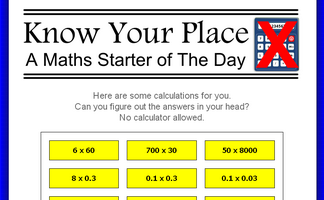Without a calculator perform some calculations requiring a knowledge of place value.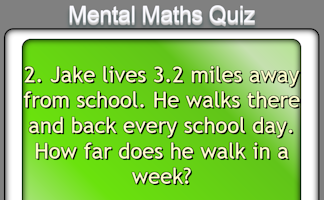A traditional twenty question mental arithmetic test presented as a PowerPoint presentation.For each pair of numbers multiply the sum by the difference then divide the answer by 5.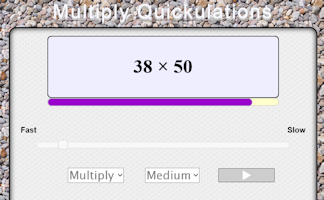Random multiplications appear on screen every few seconds.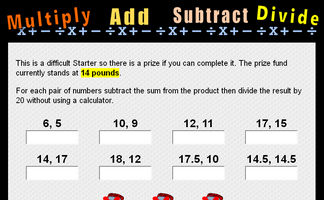For each pair of numbers subtract the sum from the product then divide the result by 20 without a calculator.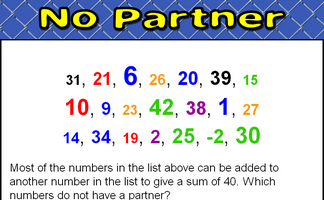Find which numbers in a given list do not combine with other numbers on the list to make a given sum.Which group of four numbers, arranged in a square, has the largest total?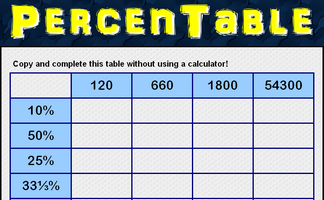Complete the table by calculating common percentages without using a calculator.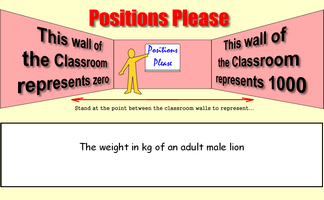Stand at the point between the classroom walls to represent a given number.It is called Refreshing Revision because every time you refresh the page you get different revision questions.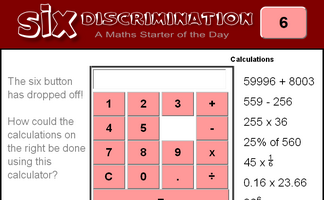An activity involving a calculator which is missing the six button. Can you evaluate the given expressions without using the six?A challenge to learn an unfamiliar times table involving decimals.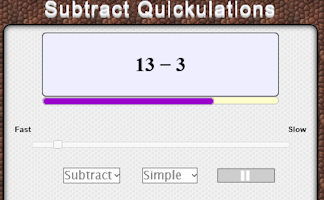Calculations appear on the screen every few seconds.Interactive number-based logic puzzle similar to those featuring in The Times and Telegraph newspapers.Learn an unusual times table from the strategic finger moving up and down the 'Table Leg'!Multiply the number on the spider's back by the numbers next to its legs.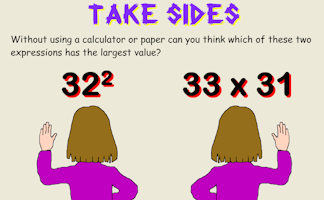Put up your right hand or left hand depending on the expressions that appears.Work out who is in which team from the information given.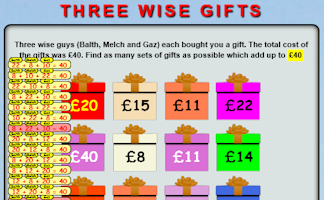Find as many sets of three of the available numbers as possible which add up to the given total.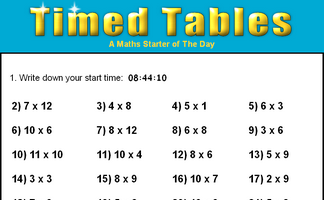How fast can you answer 24 mixed times tables questions?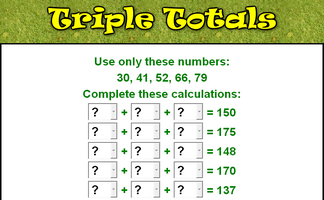Complete the sums using only the given numbers then check your calculations are correct.Find as many sets of three of the available numbers as possible which add up to the given total.Find five integers that multiply together to give a product of twelve.

### Search

The activity you are looking for may have been classified in a different way from the way you were expecting. You can search the whole of Transum Maths by using the box below.

Have today's Starter of the Day as your default homepage. Copy the URL below then select
Tools > Internet Options (Internet Explorer) then paste the URL into the homepage field.

Set as your homepage (if you are using Internet Explorer)

Do you have any comments? It is always useful to receive feedback and helps make this free resource even more useful for those learning Mathematics anywhere in the world. Click here to enter your comments.For All: